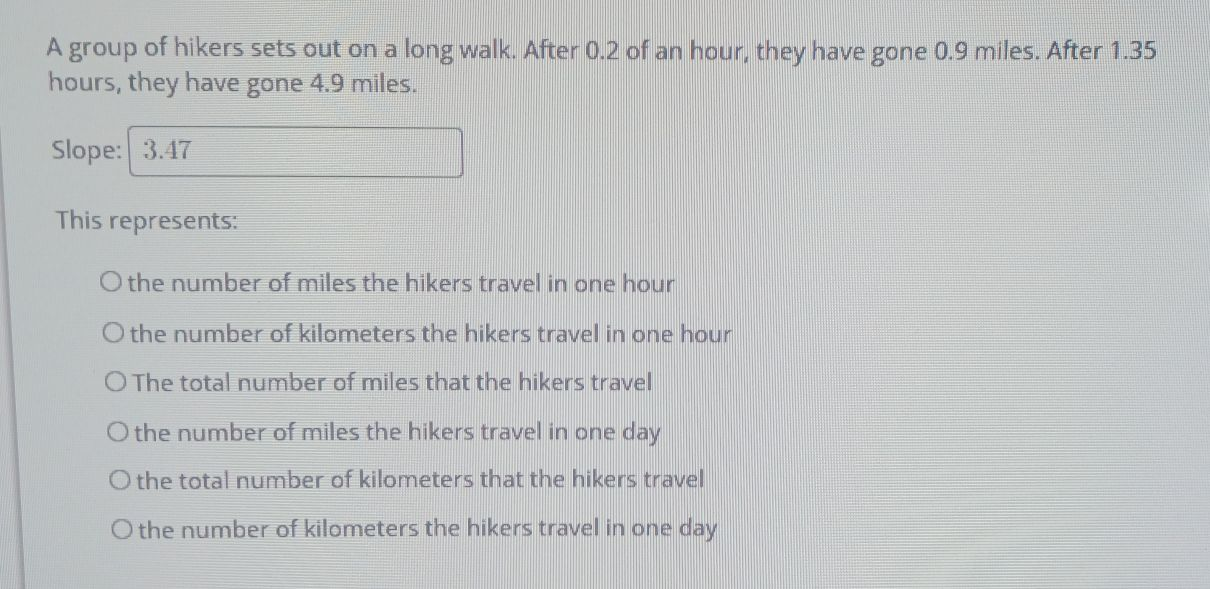### ¿Todavía tienes preguntas de matemáticas?

Pregunte a nuestros tutores expertos
Algebra
PreguntaA group of hikers sets out on a long walk. After $$0.2$$ of an hour, they have gone $$0.9$$ miles. After $$1.35$$ hours, they have gone $$4.9$$ miles. Slope: $$3.47$$

This represents:

the number of miles the hikers travel in one hour

the number of kilometers the hikers travel in one hour

The total number of miles that the hikers travel

the number of miles the hikers travel in one day

the total number of kilometers that the hikers travel

the number of kilometers the hikers travel in one day